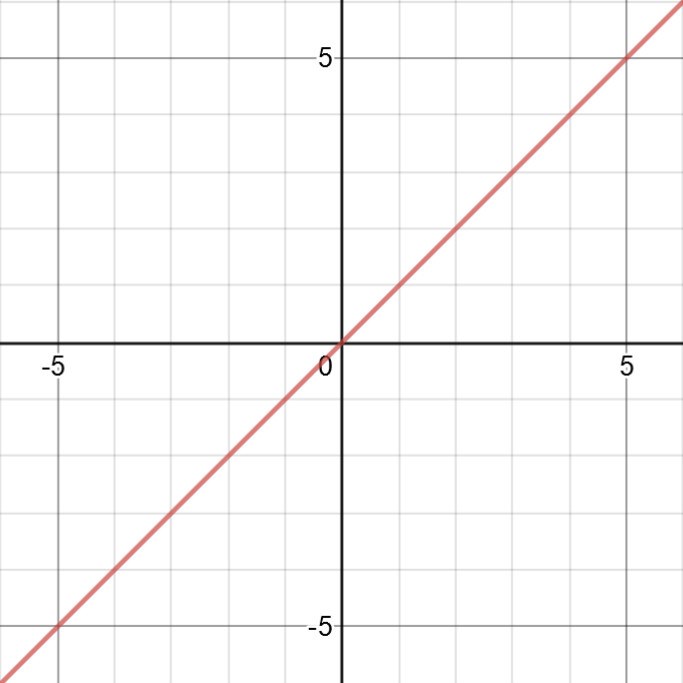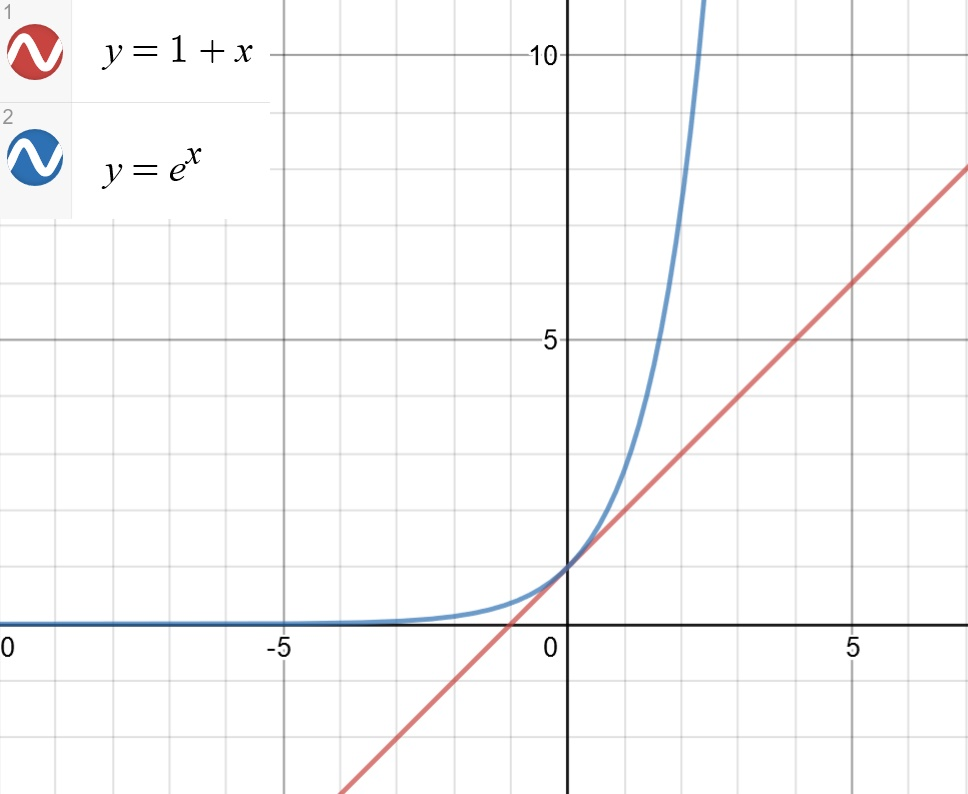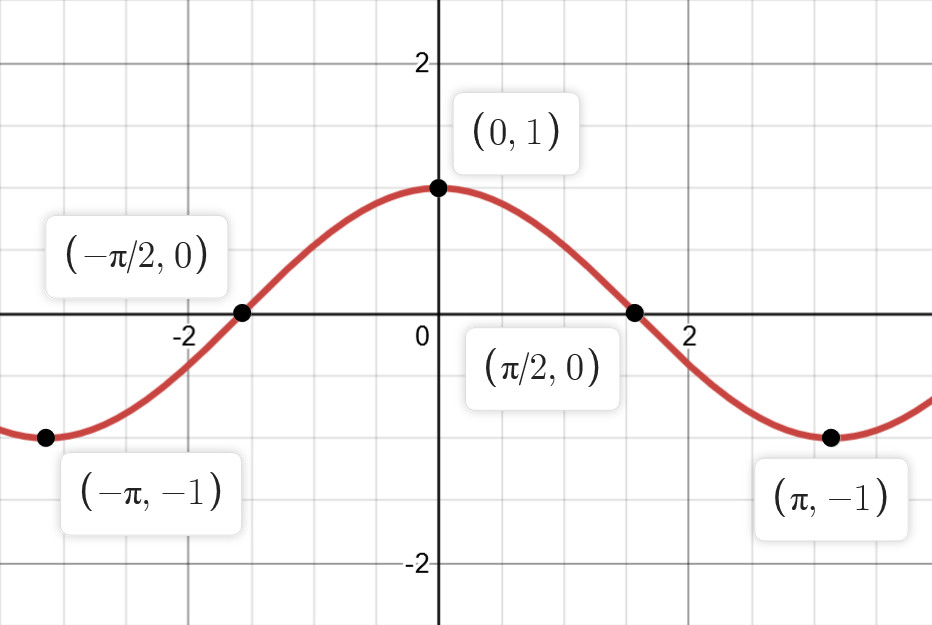## 解析

(1) 当 $\lambda_{1},\lambda_{2}$ 为互异实根时，微分方程得通解为 $y(x)=C_{1}e^{\lambda_{1}x}+C_{2}e^{\lambda_{2}x};$

(2) 当 $\lambda_{1}=\lambda_{2}$ 时，通解为 $y(x)=(C_{1}+C_{2}x)e^{\lambda_{1}x};$

(3) 当 $\lambda=\alpha \pm i \beta$ （复数根）时，通解为 $y(x)=e^{\alpha x}(C_{1}\cos \beta x+C_{2}\sin \beta x).$

$\lambda^{2}+2\lambda+3=0.$ (1)

$x=\frac{-b\pm\sqrt{b^{2}-4ac}}{2a}.$

$\lambda=\frac{-2\pm\sqrt{4-12}}{2}=\frac{-2\pm\sqrt{-8}}{2}.$ (2)

$i^{2}=-1.$

$\lambda=\frac{-2\pm\sqrt{8i^{2}}}{2}=\frac{-2\pm i 2 \sqrt{2}}{2}=-1\pm i\sqrt{2}.$

$y=e^{-x}(C_{1}\cos \sqrt{2}x+C_{2}\sin\sqrt{2}x)$

EOF

## 解析

$xy'+y=0$ 得：

$(xy)'=0.$

$xy=C \Rightarrow y=\frac{C}{x}.$

EOF

## 题目

( A ) 当 $\sum_{n=1}^{\infty}b_{n}$ 收敛时，$\sum_{n=1}^{\infty}a_{n}b_{n}$ 收敛.

( B ) 当 $\sum_{n=1}^{\infty}b_{n}$ 发散时，$\sum_{n=1}^{\infty}a_{n}b_{n}$ 发散.

( C ) 当 $\sum_{n=1}^{\infty}|b_{n}|$ 收敛时，$\sum_{n=1}^{\infty}a_{n}^{2}b_{n}^{2}$ 收敛.

( D ) 当 $\sum_{n=1}^{\infty}|b_{n}|$ 发散时，$\sum_{n=1}^{\infty}a_{n}^{2}b_{n}^{2}$ 发散.

## 解析

### 方法一：反例法

A 项：

$a_{n}=b_{n}=(-1)^{n-1}\frac{1}{\sqrt{n}}.$

$u_{n} \geqslant u_{n+1},(n = 1,2,3, \dotsc);$

$\lim u_{n} = 0,$

$p$ 级数 $\sum_{n=1}^{\infty}\frac{1}{n^{p}}\left\{\begin{matrix} 收敛 & p>1,\\ 发散 & p \leqslant 1. \end{matrix}\right.$

B 项：

$a_{n}=b_{n}=\frac{1}{n}$, 则

$\sum_{n=1}^{\infty}a_{n}b_{n}=\sum_{n=1}^{\infty}\frac{1}{n^{2}}.$

D 项：

### 方法二：用级数收敛的必要条件推导证明

$\sum_{n=1}^{\infty}u_{n}$ 收敛 $\Rightarrow \lim_{n \rightarrow \infty} u_{n}=0;$

$\lim_{n \rightarrow \infty} u_{n}=0 \nRightarrow \sum_{n=1}^{\infty}u_{n}$ 收敛。

$a_{n}^{2}b_{n}^{2} \leqslant M^{2}b_{n}^{2}.$

$\lim_{n \rightarrow \infty}|b_{n}|=0.$

$c$ 为非零常数，则 $\sum_{n=1}^{\infty}u_{n}$$\sum_{n=1}^{\infty}cu_{n}$ 具有相同的敛散性。

$\lim_{n=1}^{\infty}M^{2}|b_{n}|=0.$

$\lim_{n \rightarrow \infty}\frac{M^{2}|b_{n}||b_{n}|}{|b_{n}|}=\lim_{n \rightarrow \infty}M^{2}|b_{n}|=\lim_{n \rightarrow \infty}\frac{M^{2}b_{n}^{2}}{|b_{n}|}=0.$

$\sum_{n=1}^{\infty}u_{n}$$\sum_{n=1}^{\infty}v_{n}$ 均为正项级数，且 $\lim_{n \rightarrow \infty}\frac{u_{n}}{v_{n}}=A(v_{n} \neq 0).$

① 若 $0 \leqslant A \leqslant +\infty$, 且 $\sum_{n=1}^{\infty}v_{n}$ 收敛，则 $\sum_{n=1}^{\infty}u_{n}$ 收敛.

② 若 $0 \leqslant A \leqslant +\infty$, 且 $\sum_{n=1}^{\infty}v_{n}$ 发散，则 $\sum_{n=1}^{\infty}u_{n}$ 发散.

$\sum_{n=1}^{\infty}{a^{2}b_{n}^{2}}$ 收敛.

EOF

## 题目

$M=\int_{-\frac{\pi}{2}}^{\frac{\pi}{2}}\frac{(1+x)^{2}}{1+x^{2}}dx,N=\int_{-\frac{\pi}{2}}^{\frac{\pi}{2}}\frac{1+x}{e^{x}},K=\int_{-\frac{\pi}{2}}^{\frac{\pi}{2}}(1+\sqrt{\cos x})dx,$则 ( )

( A ) $M>N>K$

( B ) $M>K>N$

( C ) $K>M>N$

( D ) $K>N>M$

## 解析

$M=\int_{-\frac{\pi}{2}}^{\frac{\pi}{2}}\frac{(1+x)^{2}}{1+x^{2}}dx=\int_{-\frac{\pi}{2}}^{\frac{\pi}{2}}\frac{1+x^{2}+2x}{1+x^{2}}dx=\int_{-\frac{\pi}{2}}^{\frac{\pi}{2}}[\frac{1+x^{2}}{1+x^{2}}+\frac{2x}{1+x^{2}}]dx=\int_{-\frac{\pi}{2}}^{\frac{\pi}{2}}[1+\frac{2x}{1+x^{2}}]dx$

$y=x,x \in (-\infty,+\infty)$ 就是一个典型的奇函数，如图 1：Figure 1. 奇函数图像，使用 www.desmos.com 制作

$f(x)=\frac{2x}{1+x^{2}}$

$\frac{2(-x)}{1+(-x)^{2}} = -\frac{2x}{1+x^{2}} \Rightarrow f(-x) = -f(x).$

$\int_{-\frac{\pi}{2}}^{\frac{\pi}{2}}\frac{2x}{1+x^{2}}dx=0.$

$M=\int_{-\frac{\pi}{2}}^{\frac{\pi}{2}}1 d x.$

$d(x^{\mu})=\mu x^{\mu-1}dx.$

$M=\int_{-\frac{\pi}{2}}^{\frac{\pi}{2}}1+\frac{d(1+x^{2})}{1+x^{2}}=\int_{-\frac{\pi}{2}}^{\frac{\pi}{2}}1+\frac{1}{1+x^{2}}d(1+x^{2}).$

$\int \frac{1}{x}dx=\ln |x| + c.$

$M=\int_{-\frac{\pi}{2}}^{\frac{\pi}{2}}1+\frac{1}{1+x^{2}}d(1+x^{2})=x+\ln |1+x^{2}| + c |_{-\frac{\pi}{2}}^{\frac{\pi}{2}}=\frac{\pi}{2}+|\ln[1+(\frac{\pi}{2})^{2}]|+c-(-\frac{\pi}{2})-|\ln[1+(-\frac{\pi}{2})^{2}]|-c=\frac{\pi}{2}+\frac{\pi}{2}=\pi.$

$\int_{a}^{b}1dx=b-a.$

$M=\int_{-\frac{\pi}{2}}^{\frac{\pi}{2}}1dx.$

$\int \frac{2x}{1-x^{2}}dx=-\int \frac{1}{1-x^{2}}=-\ln |1-x^{2}| +c;$

$\int \frac{2x}{1-x^{2}}dx=\int(\frac{1}{1-x}-\frac{1}{1+x})dx = -\ln|x-1|-\ln|x+1|+c=-\ln|x^{2}-1|+c.$

$设 f(x) \leqslant g(x),x \in [a,b], 则 \int_{a}^{b}f(x)dx \leqslant \int_{a}^{b}g(x)dx.$

$x=0$ 时，$1+x=e^{x}=1;$

$x<0$ 时，$e^{x}$ 的减小速度小于 $1+x$ 的减小速度；

$x>0$ 时，$e^{x}$ 的增长速度大于 $1+x$ 的增长速度。Figure 2. 两个函数的对比图像，使用 www.desmos.com 制作Figure 3. 余弦函数的图像，使用 www.desmos.com 制作

$K \geqslant M \geqslant N,$ 正确选项是：C

EOF

## 题目

( A ) 若 $\{x_{n}\}$ 收敛，则 $\{f(x_{n})\}$ 收敛.

( B ) 若 $\{x_{n}\}$ 单调，则 $\{f(x_{n})\}$ 收敛.

( C ) 若 $\{f(x_{n})\}$ 收敛，则 $\{x_{n}\}$ 收敛.

( D ) 若 $\{f(x_{n})\}$ 单调，则 $\{x_{n}\}$ 收敛.

## 解析

$\{1,-1,1,-1,1,-1\}.$

• 复合函数的单调性

“同增异减”的含义就是，如果外层函数是增函数，则复合函数的增减性与内函数的增减性一致；

“同增异减”也可以理解成，如果复合前两个函数都为增函数或者都为减函数，则复合函数为增函数；如果复合前两个函数一个为增函数，一个为减函数，则复合函数为减函数。

• 复合函数的奇偶性

① 如果内函数为奇函数，则复合函数的奇偶性与外函数的奇偶性保持一致；

② 如果内函数为偶函数，则复合函数必为偶函数。

• 复合函数的周期性

① 若内函数为周期函数，则复合函数一定也是周期函数；

② 若外函数为周期函数，则复合函数不一定为周期函数。

• 复合函数的有界性

① 若内函数有界且外函数有界，则复合函数一定有界；

② 若内函数无界但外函数有界，则复合函数一定有界；

（上述两条总结一下就是，无论内函数是否有界，只要外函数有界，则复合函数一定有界。）

③ 若内函数有界但外函数无界或者内外函数都无界，这种情况下不能确定或者否定复合函数是有界还是无界，如果要确定或否定，还需要其他条件辅助分析。

A 项：

$\{x_{n}\}$ 收敛 → $\{x_{n}\}$ 有界；

$f(x)$ 有界 + $\{x_{n}\}$ 有界 → $\{f(x_{n})\}$ 有界；

A 项错误。

B 项：

$\{x_{n}\}$ 单调 + $f(x_{n})$ 单调 → $\{f(x_{n})\}$ 单调；

$f(x_{n})$ 有界 → $\{f(x_{n})\}$ 有界；

$\{f(x_{n})\}$单调有界 → $\{f(x_{n})\}$ 收敛。

B 项正确。

C 项：

C 项错误。

D 项：

$f(x_{n})$ 有界 → $\{f(x_{n})\}$ 有界；

$\{f(x_{n})\}$单调有界 → $\{f(x_{n})\}$ 收敛；

D 项错误。

EOF

## 2018 年研究生入学考试数学一选择题第 3 题解析

$\sum_{n=0}^{\infty}(-1)^{n}\frac{2n+3}{(2n+1)!}=$__

( A ) $\sin 1+\cos 1.$

( B ) $2\sin 1 + \cos 1.$

( C ) $2 \sin 1 + 2 \cos 1.$

( D ) $2 \sin 1 + 3 \cos 1.$

$\sum_{n=0}^{\infty}(-1)^{n}\frac{2n+3}{(2n+1)!}=\sum_{n=0}^{\infty}(-1)^{n}\frac{2n+1+2}{(2n+1)!}=\sum_{n=0}^{\infty}(-1)^{n}\frac{2n+1}{(2n+1)!}+\sum_{n=0}^{\infty}(-1)^{n}\frac{2}{(2n+1)!}=\sum_{n=0}^{\infty}(-1)^{n}\frac{1}{(2n)!}+2 \sum_{n=0}^{\infty}(-1)^{n}\frac{1}{(2n+1)!}.$

$\sin x = \sum_{n=0}^{\infty}(-1)^{n}\frac{x^{2n+1}}{(2n+1)!},x \in R;$ $\cos x = \sum_{n=0}^{\infty}(-1)^{n}\frac{x^{2n}}{(2n)!},x \in R.$

$\sin x = \sum_{n=0}^{\infty}(-1)^{n}\frac{1}{(2n+1)!}$ $\cos x = \sum_{n=0}^{\infty}(-1)^{n}\frac{1}{(2n)!}$

$原式 = \cos 1 + 2 \sin 1 = 2 \sin 1 + \cos 1.$

EOF